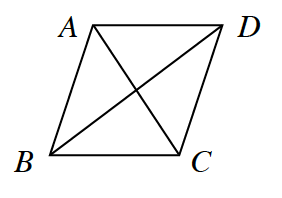### Home > CCG > Chapter 9 > Lesson 9.2.1 > Problem9-68

9-68.Examine the diagram of $ABCD$ at right.

1. If opposite sides of the quadrilateral are parallel and all sides are congruent, what type of quadrilateral is $ABCD$?

Think about the requirements for different shapes. What shape has these requirements?

2. List what you know about the diagonals of $ABCD$.

Look at the diagram. What do these lines do?

They are perpendicular and intersect each other at their midpoints. They also bisect the angles of the quadrilateral.

3. Find the area of $ABCD$ if $BC = 8$ and $m∠ABC = 60°$.

Find the area of one of the triangles, then find the total area. What do you know about the triangles that can help you?

The angles at the center are $90°$, so it is a $30°\text{-}60°\text{-}90°$ triangle.

The length from the angle to the center is $4\sqrt{3}$.

Triangle: $\frac{1}{2}(4)(4\sqrt{3})=8\sqrt{3}$

Total Area: $4(8\sqrt{3})$

$55.4\text{ units}^2$